# Get Real with Wolfram|Alpha—Computing Roots

April 26, 2013 —Comments Off

Some common questions from the many student users of Wolfram|Alpha include “Isn’t cbrt(-8) = -2?” and “Why doesn’t the plot of the cube root include the negative part?” The answers are that -2 is just one of the three cube roots of -8, and that Mathematica, the computational engine of Wolfram|Alpha, has always chosen the principal root, which is complex valued. More generally, odd roots of negative numbers are typically assumed to be complex. You can see this in the output of (-8)^(1/3).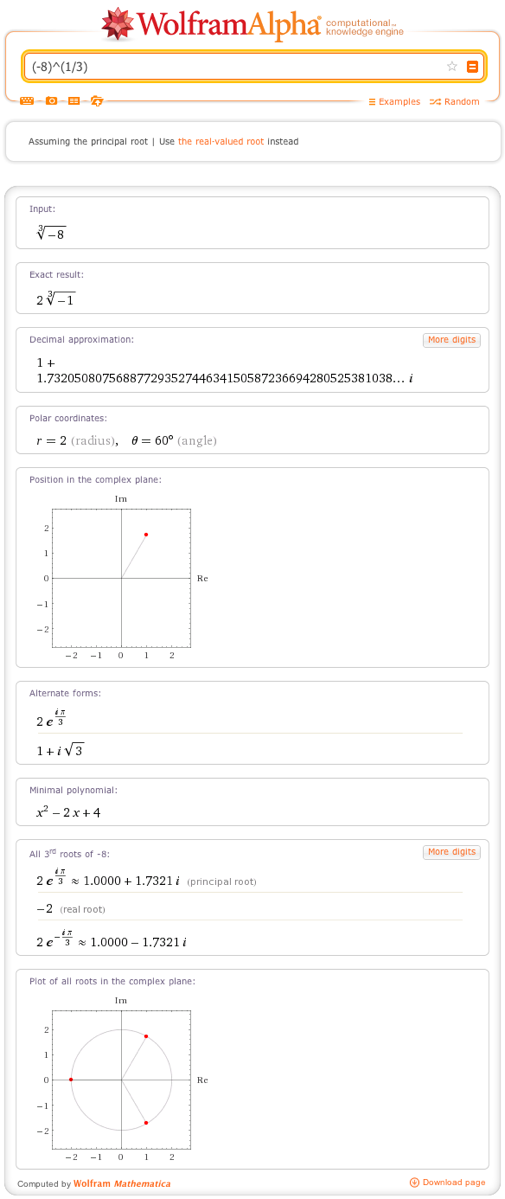Note that the principal value of about 1 + 0.723i is indeed a complex number. In addition, all 3rd roots of -8 are displayed, as are their positions in the complex plane.

It should be mentioned that there are very good reasons to use the principal root in higher mathematics, as this is considered to be the proper way to extend the root functions into the complex number system. With the release of Version 9 of Mathematica, however, there are also new, real-valued functions for computing roots, and these are incorporated naturally into Wolfram|Alpha. One way to access these new functions is to select the “Use the real-valued root instead” option below the input bar as shown in the previous example. Alternatively, we can access Mathematica‘s CubeRoot function, for example, by typing “cube root” instead of using the power ^(1/3), as in the previous example.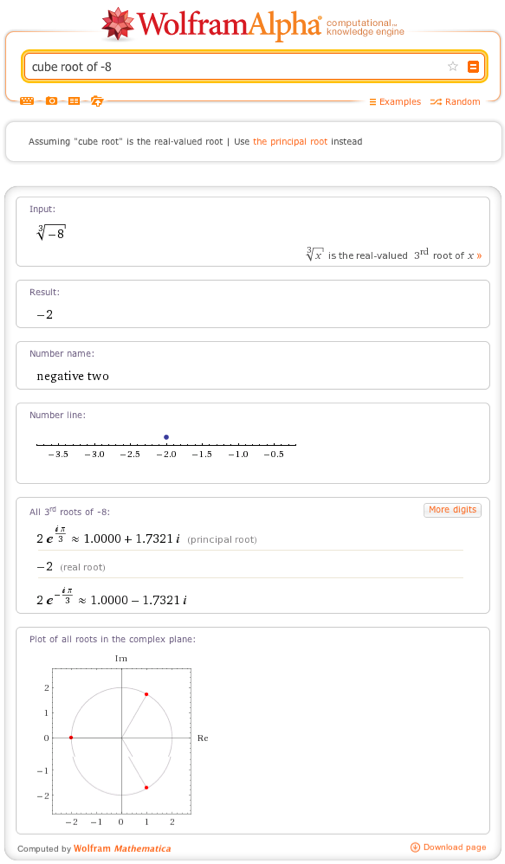Note that the result is, indeed, -2, and that some of the information specific to complex numbers is missing. We do still receive the information indicating how this relates to all the roots, however. A shorthand way to enter the real cube root is “cbrt”. We can obtain a lot of information on this function like so: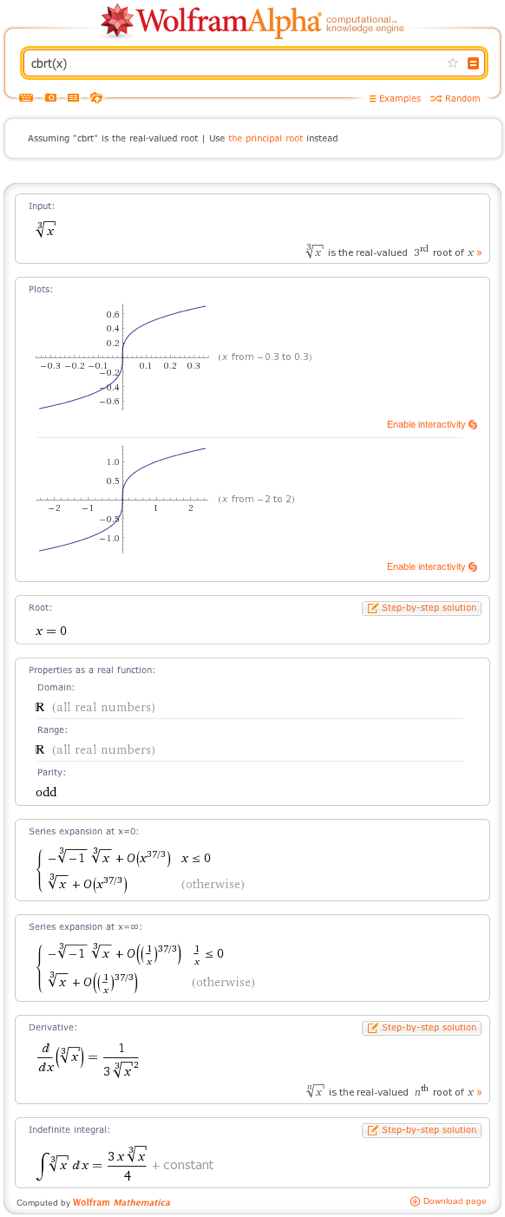Note that the plots extend over the whole real line, and that the domain and range computations reflect this. Of course, we can access other odd roots as well. For example, we might specify that we want the real-valued 11th root of -12.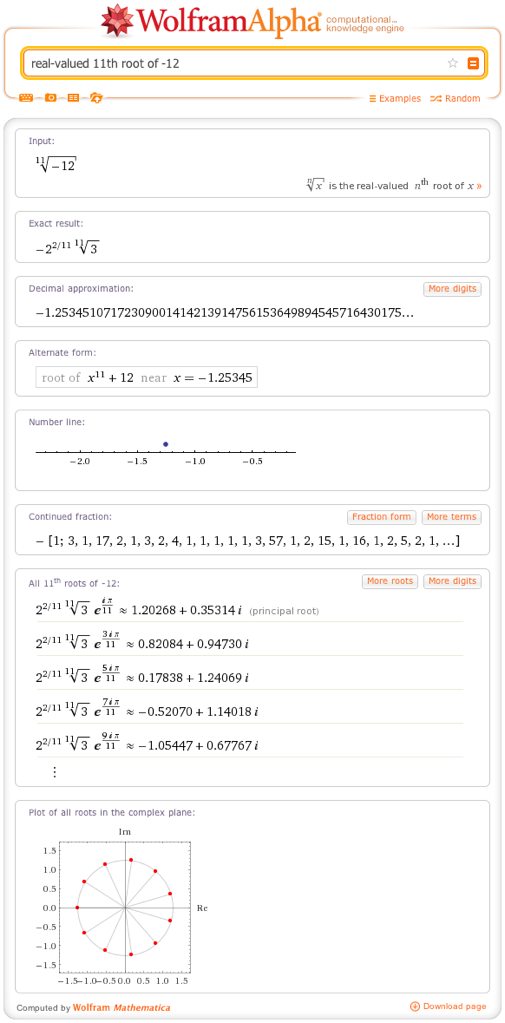Just as with cube roots, there’s a handy shorthand called surd. This is particularly useful if you want to use a real root as part of a more complicated computation, as in our final example.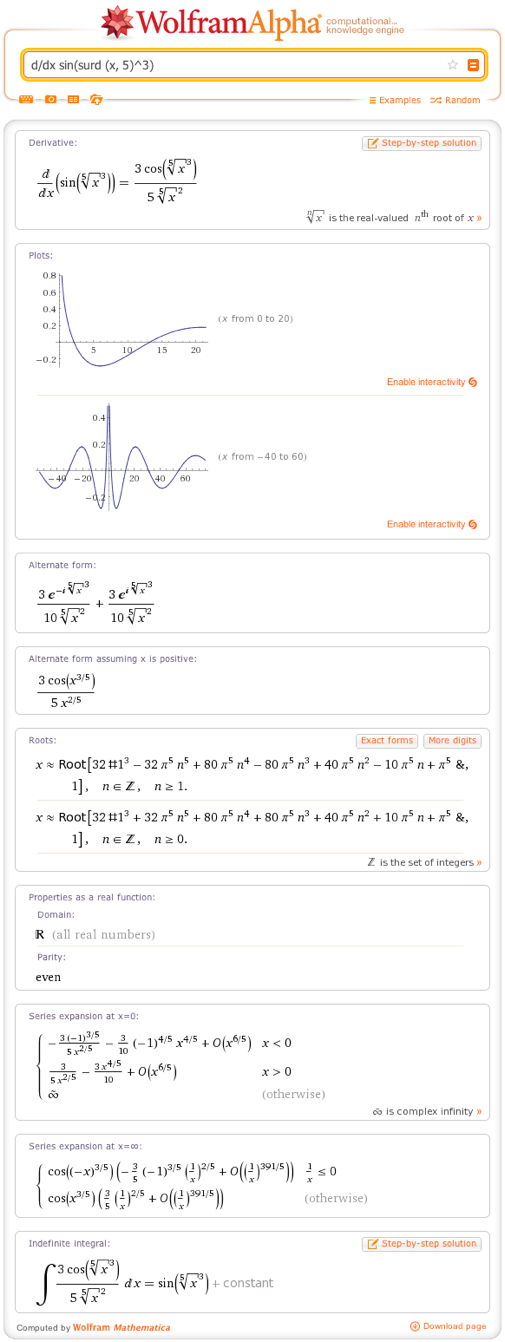We hope you like this new functionality, and keep it real!